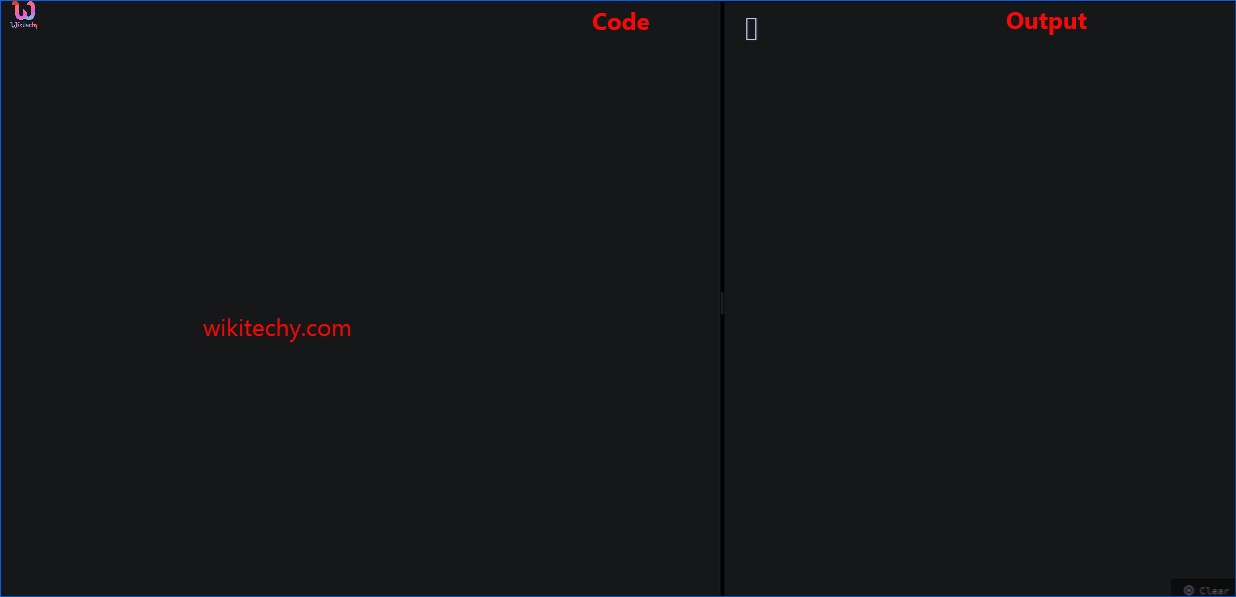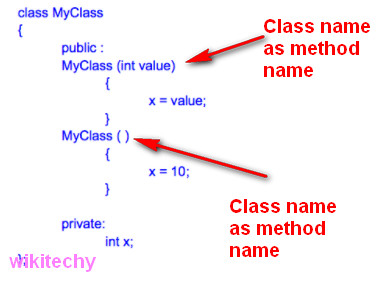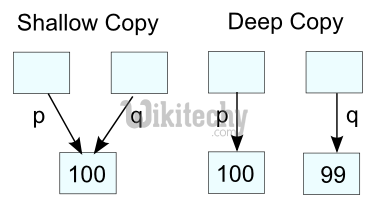# Constructors C++ | C++ Constructors - Learn C++ - C++ Tutorial - C++ programmingLearn c++ - c++ tutorial - c++-constructors - c++ examples - c++ programs

## What is Constructor in C++?

• A constructor is a special method of a class or structure in object-oriented programming that initializes an object of that type.
• A constructor is an instance method that usually has the same name as the class, and can be used to set the values of the members of an object, either to default or to user-defined values.
• Constructors are special type of member functions that initializes an object automatically when it is created.
• Compiler identifies a given member function is a constructor or not by its name and the return type.
•learn c++ tutorials - constructor in c++ Example

• Constructor has the same name as that of the class and it does not have any return type. Also, the constructor is always being public.
• The below program shows a constructor is defined without a return type and the same name as the class.

## How constructor works?

• In the above pseudo code, temporary() is a constructor.
• When an object of class temporary is created, the constructor is called automatically, and x is initialized to 5 and y is initialized to 5.5.
• You can also initialize the data members inside the constructor's body as below. However, this method is not preferred.

## Use of Constructor in C++

• Suppose you are working on 100's of Person objects and the default value of a data member age is 0. Initializing all objects manually will be a very tedious task.
• Instead, you can define a constructor that initializes age to 0. Then, all you have to do is create a Person object and the constructor will automatically initialize the age.
• These situations arise frequently while handling array of objects.
• Also, if you want to execute some code immediately after an object is created, you can place the code inside the body of the constructor.

## Example 1: Constructor in C++

• Calculate the area of a rectangle and display it.
• Calculate the area of a rectangle and display it.
• In this program, class Area is created to handle area related functionalities. It has two data members length and breadth.
• A constructor is defined which initialises length to 5 and breadth to 2.
• We also have three additional member functions GetLength(), AreaCalculation() and DisplayArea() to get length from the user, calculate the area and display the area respectively.
• When, objects A1 and A2 are created, the length and breadth of both objects are initialized to 5 and 2 respectively, because of the constructor.
• Then, the member function GetLength() is invoked which takes the value of length andbreadth from the user for object A1. This changes the length and breadth of the object A1.
• Then, the area for the object A1 is calculated and stored in variable temp by callingAreaCalculation() function and finally, it is displayed.
• For object A2, no data is asked from the user. So, the length and breadth remains 5 and 2 respectively.
• Then, the area for A2 is calculated and displayed which is 10.

## Output

• Overloaded constructors have the same name (name of the class) but different number of arguments.
• Depending upon the number and type of arguments passed, specific constructor is called.
• Since, there are multiple constructors present, argument to the constructor should also be passed while creating an object.
###### Learn C++ , C++ Tutorial , C++ programming - C++ Language -Cplusplus

• For object A1, no argument is passed while creating the object.
• Thus, the constructor with no argument is invoked which initializes length to 5 and breadth to 2. Hence, area of the object A1 will be 10.
• For object A2, 2 and 1 are passed as arguments while creating the object.
• Thus, the constructor with two arguments is invoked which initializes length to l (2 in this case) and breadth to b (1 in this case). Hence, area of the object A2 will be 2.

## Default Copy Constructor

• An object can be initialized with another object of same type. This is same as copying the contents of a class to another class.
•learn c++ tutorials - copy constructor in c++ Example

• In the above program, if you want to initialize an object A3 so that it contains same values as A2, this can be performed as:
• You need to create a new constructor to perform this task. But, no additional constructor is needed.
• This is because the copy constructor is already built into all classes by default.